# Workflow: Creating free form theater curtain Using Dynamo and Revit 2016

Today we will see how to use the conceptual design in Dynamo to create a Free Form Theater Curtain.
The curtain is a surface which is lofted from several lines. Each line is the connection of two points the one at the bottom and the one at the top.
The points at the bottom are following the Sin wave shape at elevation zero, while the top points are following a straight line at height 1000.
By changing the slider you can control the amplitude of the sin wave hence the deformation of the curtain.
To implement the workflow we need the following Nodes:
• 4 x Number
• Range
• Integer Slider
• Math.Sin
• 2 x Points.ByCoordinates
• Line.ByStartPointEndPoint
• Surface.ByLoft
• ImportInstance.ByGeometries

From the the “Range” Node we can get a set of points which will be incorporated to represent the start and end points. As the end points are composing a straight line we will use them as they are. But the start points are composing a Sin wave shaped curve so we need to convert their Y values first to degrees and hence use them as values to the angle input in the “Math.Sin” Node.

Once we got the set of start points and end points we will use the “Line.ByStartPointEndPoints” Node to create the lines which will be used to create the surface.

The “Surface.ByLoft” Node will create the surface based on the supplied lines by lofting. Final step is to import the geometry into Revit and this is done by using the “ImportInstance.ByGeometries” Node.# Workflow: Create Topography from DWG in Revit 2016 using Dynamo

Today we will see how to create a Toposurface in Revit from DWG file coming from Civil 3D and contains the contour lines.
When the CAD is inserted you may need to do some cleanup like removing text layers, annotation layers, grids or any layer except the contour lines layer.
When the DWG is ready, use the following workflow to create the topography.Simply click the select button and from the Revit view select the imported DWG instance. Dynamo will extract the contour lines after exploding the DWG geometry. To control the accuracy of the contour lines we shall divide the curves coming out from the explode command into arbitrary number of segments (controlled by the “Integer Slider” Node).
To create the surface we need points. The points can be generated from the segmented lines by getting the points at the middle of each segment.By clicking “Run” we get our desired Toposurface.# Workflow: Family Distribution Part 3

In today’s lesson we will see how to distribute the “Chair” Family over a squared equally spaced grid.

The lacing technique will be used in this lesson to quickly establish the square grid of eleven rows and eleven columns spaced equally by 1000 unit.

We will need to use the following Nodes:

• 4 x Number
• Range
• Family Types
• Point.ByCoordinates
• FamilyInstance.ByPoint

First we will generate a list of X and Y values by using the “Range” Node. These values will specify the number of rows, columns and the distances between them respectively.

By changing the lacing of the “Point.ByCoordinates” Node to Cross Product, we get every combination between each value in the X values list and Y values list, giving us an 11 x 11 grid of points. This is an equivalent data structure to the cross product, except our data is now a list of lists.

From the “Family Types” Node select the “Chair” Family. By connecting the “FamilyInstance.ByPoint” Node input to the “Family Types” Node output and the “Point.ByCordinates Node output, we can see that we have 121 chairs arranged in a grid of 11 rows by 11 columns.# Workflow: Family Distribution Part 2

In the second part of the “Family Distribution” lesson we will use the Family “Chair” and distribute it on a closed path around a Table.

In the part 1 of this lesson we allowed Dynamo to calculate automatically the length of each segment by dividing the total length of the path by the number of segments. This time we will do the opposite, we will specify the segment length and let Dynamo calculate the number of segments needed.

For this part of the lesson we will draw a Model Curve on the shape of a circle to use it as a guide for the distribution path, divide it into equally spaced segments then insert the “Chair” Family at the end point of each segment. We will let the “Table” Family be at the center of the circle.

So as we planned the workflow logic in our mind we can find the nodes that are needed to implement it. Now we will open Dynamo and create a new Workspace.

From the Categories Search find the following nodes:

• Select model element
• Element.Curves
• Curve.DivideByDistance
• Number Slider
• Curve.EndPoint
• Family Types
• FamilyInstance.ByPoint

In order to insert a model element from Revit environment into Dynamo environment we shall use the ”Select model element” Node, so click on select and from Revit project pick the model line.

After the Model Curve is brought into Dynamo we need to extract the Curve geometry from it so we connect the ”Select model element” Node output to the “Element.Curves” Node input. The curve geometry is ready now to be divided so we will connect the output of the “Element.Curves” Node to the input of the “Curve.Divide Equally” Node input.

To determine the length of each segment we will connect the “Number Slider” Node to the “divisions” input in the “Curve. DivideByDistance” Node. By changing the slider value the number of divisions will increase or decrease respectively.

As the curve has been segmented we need to locate the end points as they will be our insertion positions for the “Chair” Family instances. So connect the output of the “Curve. DivideByDistance” Node to the “Curve.EndPoint” Node input. Now from the “Family Types” Node select the “Chair” Family Type and connect it to the “FamilyInstance.ByPoint” Node.

The last step before hitting the “Run” button is to connect the insertion points coming from the “Curve.EndPoint” Node into the “FamilyInstance.ByPoint” Node. We can hit “Run” button and see the result in the Revit view. If we set the run mode in Dynamo to automatic then we can change the number of inserted instances based on the length of segments by changing the value of the Number Slider online.The Dynamo Workflow can be downloaded from here# Workflow: Family Distribution Part 1

Today we will talk about how to distribute instances of a Revit Family uniformly along a predefined path using Dynamo 0.9.2 in Revit 2016. The path could be either closed or open.

First open Revit and make sure that the Family you want to distribute is loaded.

In our example we will use the Family “Chair” and distribute it once along a curved line.

For this lesson we will draw a model curve to use it as a guide for the distribution path, divide it into equally spaced segments then insert the “Chair” Family at the end point of each segment.

So as we planned the workflow logic in our mind we can find the nodes that are needed to implement it. Now we will open Dynamo and create a new Workspace. From the Categories Search find the following nodes:

• Select model element
• Curves
• Divide Equally
• Number Slider
• EndPoint
• Family Types
• ByPoint

In order to insert a model element  from Revit environment into Dynamo environment we shall use the ”Select model element” Node, so click on select and from Revit project pick the model line.

After the Model Curve is brought into Dynamo we need to extract the Curve geometry from it so we connect the ”Select model element” Node output to the “Element.Curves” Node input.

The curve geometry is ready now to be divided so we will connect the output of the “Element.Curves” Node to the input of the “Curve.Divide Equally” Node input.

To determine the number of segments needed we will connect the “Number Slider” Node to the “divisions” input in the “Curve.Divide Equally” Node.  By changing the slider value the number of divisions will increase or decrease respectively.

As the curve has been segmented we need to locate the end points as they will be our insertion positions for the “Chair” Family instances. So connect the output of the “Curve.Divide Equally” Node to the “Curve.EndPoint” Node input.

Now from the “Family Types” Node select the “Chair” Family Type and connect it to the “FamilyInstance.ByPoint” Node.

The last step before hitting the “Run” button is to connect the insertion points coming from the “Curve.EndPoint” Node into the “FamilyInstance.ByPoint” Node.

We can hit “Run” button and see the result in the Revit view. If we set the run mode in Dynamo to automatic then we can change the number of inserted instances based on the number of segments by changing the value of the Number Slider online.# Workflow: SRTM Data to Topography in Revit

Overview: This workflow allows the user to source SRTM Data in GeoTIFF format from http://earthexplorer.usgs.gov/, and use it to create topography in Revit. Shuttle Radar Topography Mission (SRTM), was carried out by Nasa in 2000 and recorded elevation data. This data has been made available globally at 1 Arc Second, and in more detail at 1/3 Arc Second for some parts of the US.

Packages: ‘Elk’.# Workflow: Renumber Doors by Guide Curve

designtech recently posted a useful workflow that automatically renumbers Doors in Revit based on a guide curve.

Read the whole post and see more images and videos at:

# Workflow: Automatically Renumber Rooms with Level Detection

This one comes via Vikram Subbaiah on the forum.

In simple terms, it will detect what level a room is on and then automatically generate and apply a suitable room number.

DYN

RVT

# Workflow Repost: Batch Create Elevation Views with Dynamo

I have posted previously about Section View creation, but here is a nice workflow from Jeremy Graham on Module 2 at the Learn Dynamo website, original post here. Great work and an excellent explanation too. Full credit to Jeremy Graham for this workflow.

Content:

The script was primarily built for model lines although it can be used for any line based family type such as walls …Select Model Elements is used to select the model elements in the project file. From these curve elements, CurveElement.Curve allows us to extract the base curve of the element into a usable Dynamo format. This is where you would need to alter the script to suit selecting walls.Once we have the curves we need, we can start extraction information from them for the script. StartPoint and CurveEndPoint both do just what they sound like ; get the endpoints of the curves. These points are then combined into a single list using List.Create and the result is transposed using List.Transpose. If you are not familiar with the concept or transpose, it is basically used to interchange the number of rows with the number of columns in a matrix or vice versa. In this case, the list of two sub lists has been transposed into a list of six sub lists based on the original number of items in the first list. This list of points has been transposed to match the number of planes coming out of Plane.XAxiz. This can be seen in the two watch nodes below where the left is before the transform and the right is after.

From the curve elements we also extract a coordinate system using Curve.CoordinateSystemAtParameter. This is set at parameter 0.5 which is the midpoint of the straight line. Using this new coordinate system, we create a plane with Plane.XAxis and convert the coordinate systems to points using Point.ByCartesianCoordinates.The list of transposed points and planes extracted in part 2 can now be moved or translated using the Translate node. The new planes will be used for the elevation markers and the curve end points will set up the boundary of the crop views. The Number Slider allows us to adjust the location of these points in relation to the model line. Putting this number in the positive or negative spectrum will adjust the marker location to either side of the model line.

A diagram of what is occurring in this section is shown below. This is based on a dodecagon shape made up my model lines.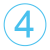Using the translated points for the crop view boundary, we once again transpose the list so that we can swap the list back to its original form of 2 items. Once it is in this form List.FirstItem and List.LastItem are used to get all the items from each sub list.

The translated points for the elevation markers will be used later when the elevations are created.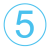Now that we have all the translated curve endpoints in two separate lists, we can use these to create new lines with the Line.ByStartPointEndPoint. These lines will make up the base of the crop views so Curve.ExtendEnd and Curve.ExtendStart nodes are added so that we can extend the crop view as needed by the value stated in the Code Block.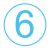From the extended curves, we once again get the start and end points from Curve.StartPoint and Curve.EndPoint respectively. A list is then made from these and transposed to achieve the same result as shown in part 2 which is the end points of each crop view in their own sub list. This can be seen below in the sample diagram.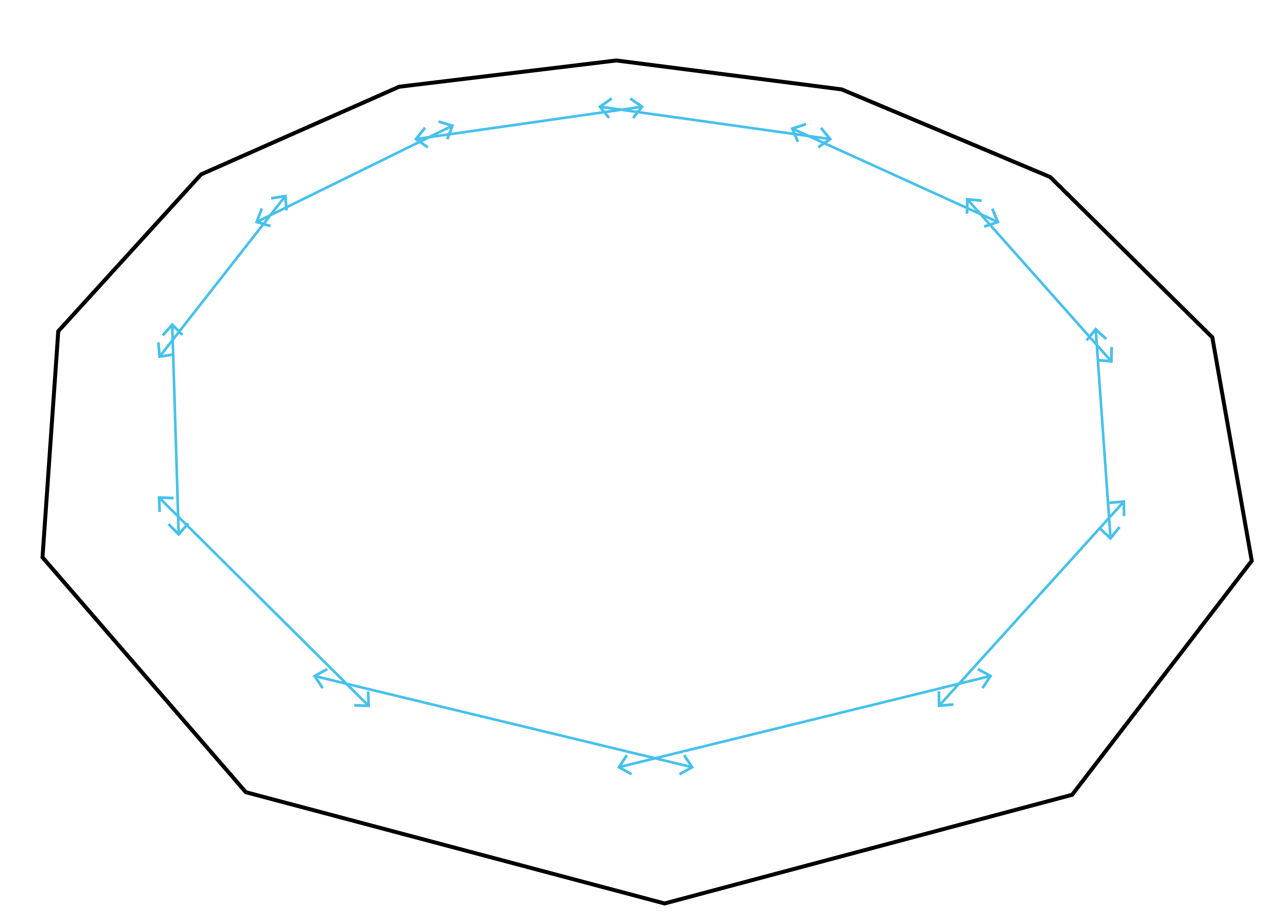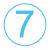Similarly to part 3, the points obtained in part 6 are translated using Geomery.Translate but this time in the Z axis using Vector.ZAxis. This creates the upper corners of the crop views that will be created and are controlled using either Number Slider to move the points up or down.Both lists from the Geometry.Translate nodes are transposed so that all end points are grouped together and in the same fashion of part 4, we gather up each of the end points using List.FirstItem and List.LastItem. All of these points now form the corners of each crop view that will be created. Therefore, they are grouped together in the List.Create node and in an anti-clockwise order.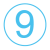List.Transpose makes a return to separate all these grouped lists into their corresponding crop views so we now have all the points of each crop view in their own sub list. From this, PolyCurve.ByPoints is used to make a loop from each sub list of points. Each curve of these loops are then extracted using PolyCurve.Curves so that they can be used in the following Python Script. The poly curves that have now been created are shown diagrammatically below.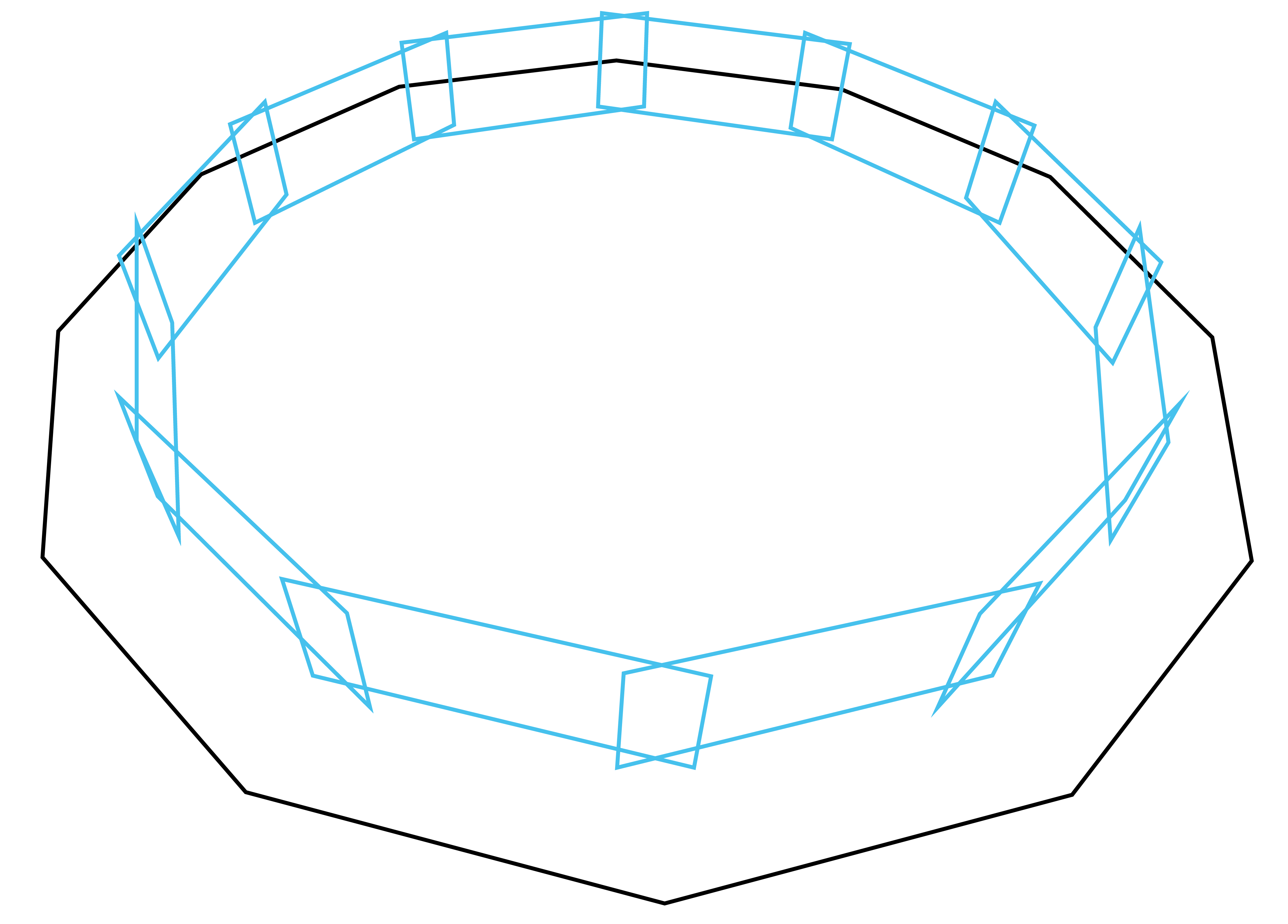Before we create the elevations, we need to select the ViewFamily Type to use. This Python Script does just that. The details of this are shown below starting at part 10.1 onwards. The output of this script is a list with two sublists, one that contains the ViewFamily Types and the other with their names. With this information, we can use the Code Block node to first select the list of elements using the  code and then the number of which element we want to use, in this case the first so another  is added.The final part of this module is completed using a Python Script. I originally thought this would be a simple script but it turned out to be quite large. It’s relatively simple to understand though so please read through to get an understanding of how it works in part 11.1 onwards.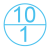With these Python Scripts I am not going to explain the importing parts as the reason for these are explained in module 1.

To start, 3 empty lists are created under the variables elements, names and lst. These will be the outputs of the script.A FilteredElementCollector is then used to filter the document (doc) of class ViewFamilyType and the result is set to the variable collector as elements.With all these elements collected, we loop through them with a for loop. In the first loop, we find View Families of the type Elevation and append it to the elements list. Then another loop is set up to loop through the parameters of this element. Once the loop finds the elements Type Name it appends this to the names list. Finally, both the elements list and names list are appended to the output list; lst.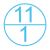The incoming data is stored under the corresponding variables. This includes the points where the elevation markers will be created (points), the midpoint of the original model lines (modelPoints), the curves that make up the crop views (cropCurves) and the ViewFamily type chosen in part 10 (viewType).As shown in module 1, the toggle is set to True to make the script activate. This is so we can refresh the script if needed. We then start a transaction which is needed to create elements within Revit, more information about this can be found here.

We then start a for loop through the points list. The enumerate function is used to get the index of each item in the list. So , we can then get the model line mid-point (modelMP) from the modelPoints list at the same index as the current point in the points list.

ToXyz converts the Dynamo point to Revit type as explained in this link and from this the X and Y values are taken from modelMP.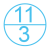Each individual curve line is taken from the cropCurves list at the same index as the current point in the points list. Each one of these curves are set to l1, l2, l3 and l4.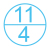The current elevation marker point is set to elevationPT. This is used to make another point (elptRotate) which is located 100 units above elevationPT in the Z axis and both points are used to make a line set to ln. This line will be used as the axis for elevation marker rotation.In this part, the X and Y values are taken from the elevationPT point and with these values, we subtract the modelMP X and Y values that we extracted earlier. These new values are then used to perform an atan2 function which gets us the angle between both points. This angle will be used to rotate the elevation marker towards the model line.With all the information gathered, we can now create an elevation marker using the document (doc), view type (viewType.Id), location point (elevationPT) and scale of view (100). An elevation is then created at that marker and set to variable ele.

The elevation marker is then rotated to be pointing at the wall using ElementTransformUtils.RotateElement(Document, element ID, rotation axis, angle).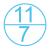The crop region manager is set to crManager and then we gather the curves brought in earlier to form a new curve loop which is then set to cLoop.Finally, we try to set the crop region of the elevation to the new curve loop using SetCropRegionShape and hopefully it is successful!

If the python script is successful then we should have a group of newly formed elevations show up in revit, all pointing towards the model line at the side set. The crop region should also be set as per the parameters in parts 3 and 7 for length and height respectively.

An example of how this should appear can be seen below. If you come across any problems, please let me know but hopefully that isn’t the case. Also, if there is anything I haven’t fully explained or you do not fully understand please give me a shout via email or in the comments below. In addition to these, if you liked the script and want more, please follow me on twitter @LearnDynamo and subscribe to the emailing list below for the next module release.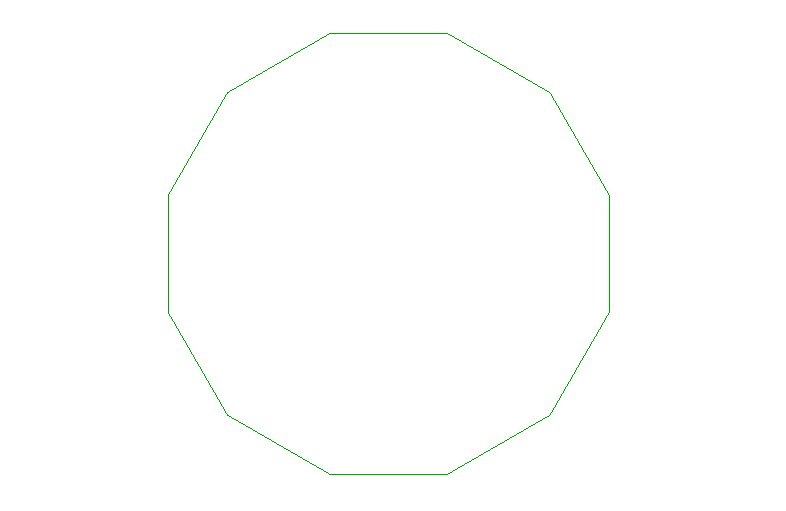# Workflow Repost: Tekla Structural Model to Excel to Revit with Dynamo## In Tekla

### 1. Manage → Organizer to open project organizer2. Open Settings to create new template3. Create new Template, create new Columns, search the following Properties:
[ Name, Number, Start_X, Start_Y, Start_Z, End_X, End _Y, End _Z, Height, Width, Profile ]4. Select all element of the model, then Export data to ExcelIn Excel

1. Save as new table sheet file, delete Row 1 ~ 4,
Sort Name Column to each new sheet2. For Position data, delete other data Column except Start and End X, Y, ZIn Dynamo

1. Create Point, Line for Structural System (girder, beam) from excel file2. Create another Point, Line for Structural Column with the same workflowDynamo nodes capture 1:
Dropbox picture1.Create a Code Block list depending on the levels.
(can just ignore the typical levels)2. Filter to divide each part from levels
(repeat this step to create each level’s filter list)

3. StructuralFraming.BeamByCurve
(do not create the entire model, do only for the non-typical levels)
(you can also filter and sort more frame lists to create different types by step.2)

4. Create the columns with the same workflowDynamo nodes capture 2:
Dropbox picture

Next time I’ll write the rest part of Number and Profile dimension linking.